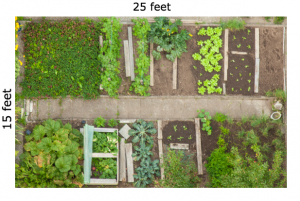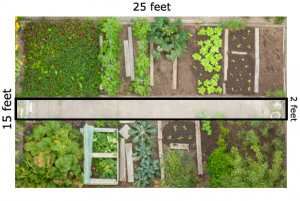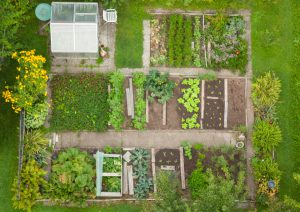Learning means building knowledge that will empower you in your future; it means understanding a concept or skill to the point where you can apply it to novel situations throughout your life. As students engage in various assignments and activities, ask yourself to what extent each will lead to building an understanding of content that will lead to application.

Let’s look at understanding. Most teaching in the past was for “procedural automaticity.” For example, what is the area of the garden below?Most people have learned that area equals length times width; so multiply 15 by 25 and you have the answer. Memorizing a procedure and applying it to similar questions is procedural automaticity; it does not require understanding.

Suppose you want to know the plantable area of the garden, how would you calculate that?If you understand the concept of area and how to calculate it, you would realize that you would find the total area of the garden and then subtract the area of the walkway in order to determine the plantable area. To figure that out, you had to understand area.

In another question, you might find a smaller garden attached to one side, thus requiring a different calculation.In my book It’s Not What You Teach But How, I introduce the term “novelity” as being the ability to apply learning to novel situations, which requires a level of understanding.

As students engage in various activities, ask yourself:

1. Am I offering “learning activities” that ensure students are receiving direct instruction and opportunities to explore concepts and skills?
2. Am I asking questions of individual students to push their level of understanding? Ex: “What if …?” “Explain how you know your answer is correct.”
3. Am I offering students very different follow-up problems to which to apply the learning?
4. Am I asking students to explain their learning?

Teach for understanding! Change the world!